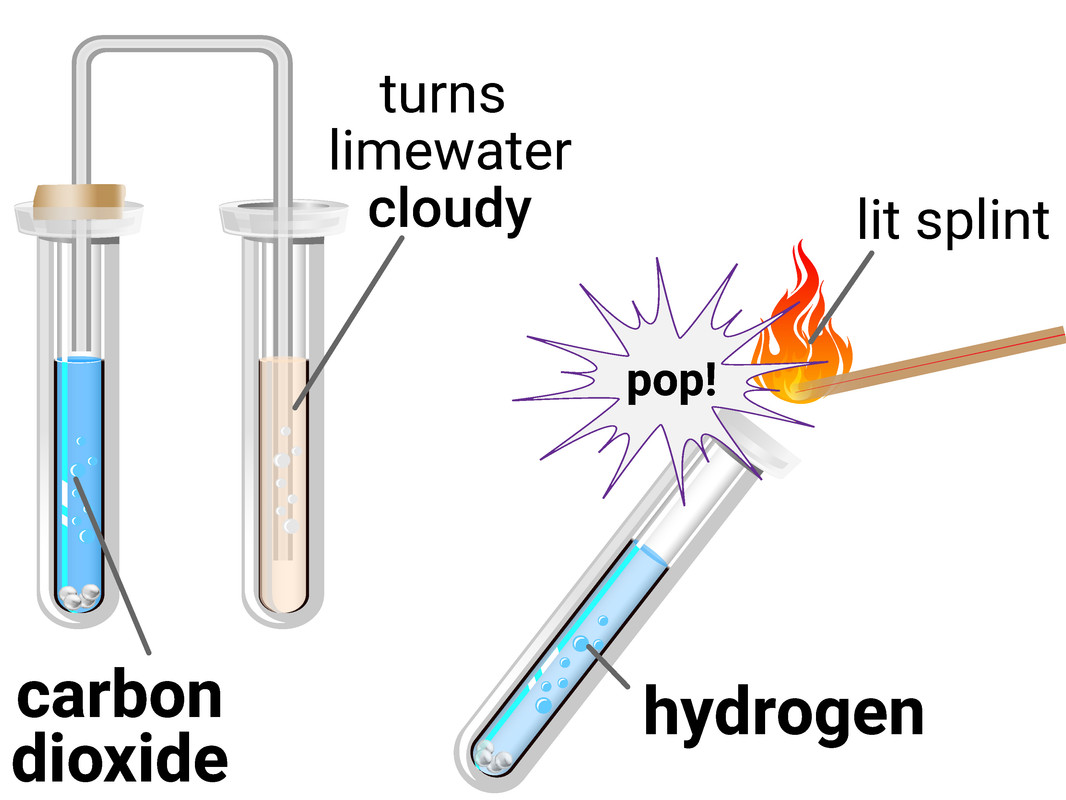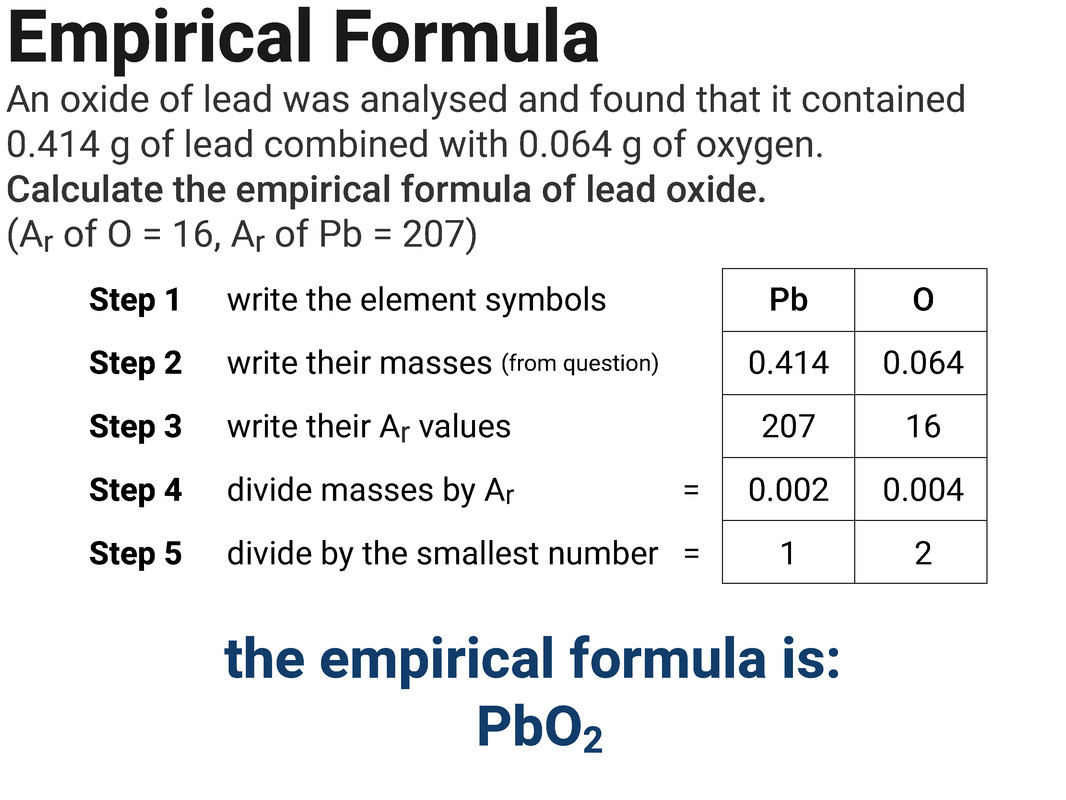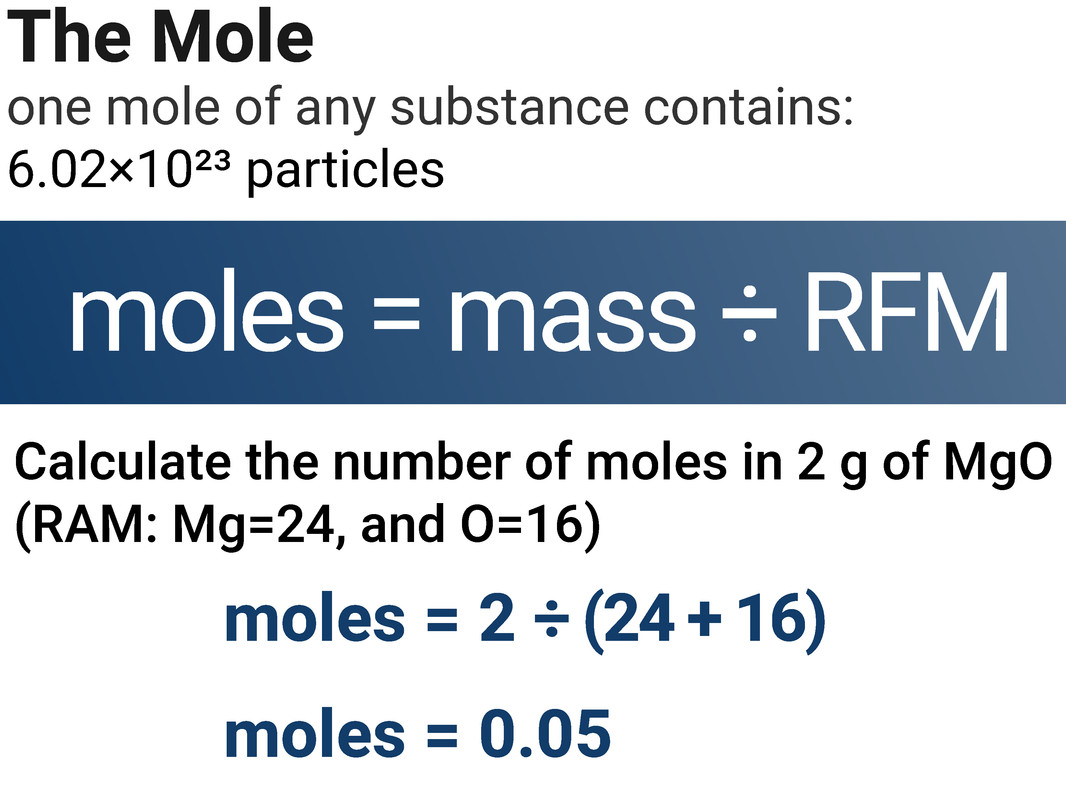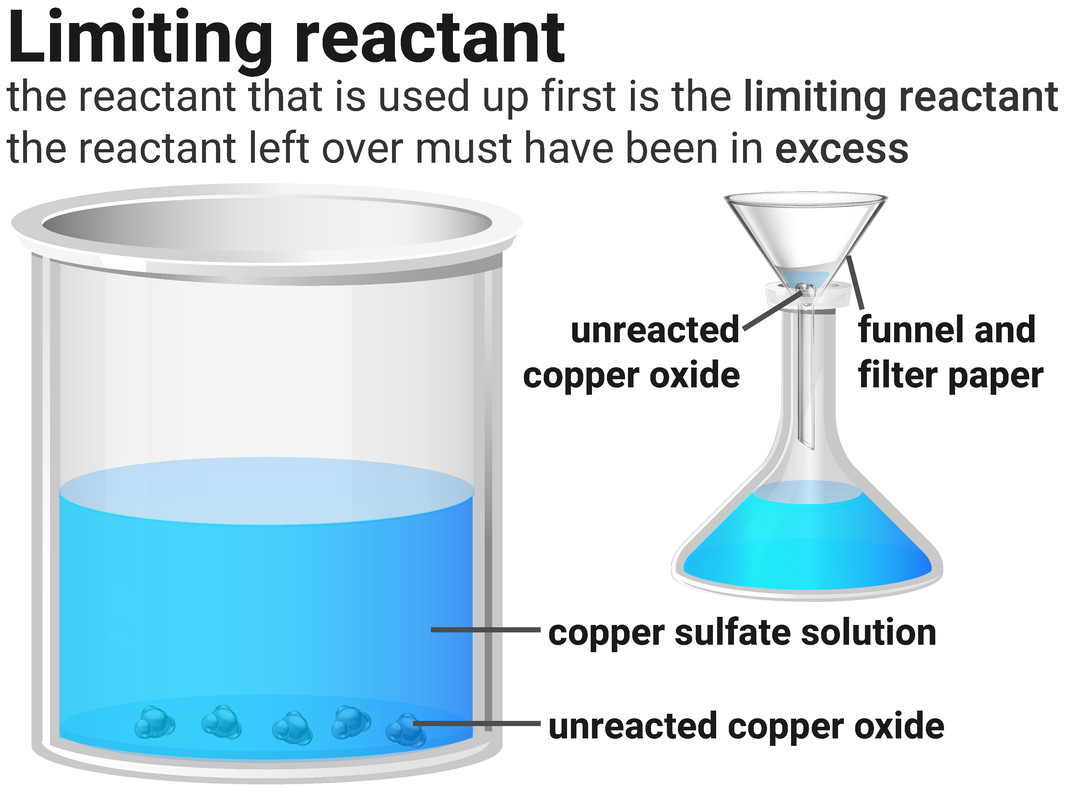# Introducing chemical reactions

### Hydrogen

The test for hydrogen uses a burning splint held at the open end of a test tube of the gas. Hydrogen burns rapidly with a pop sound.

This test requires you to allow a second, or two, for oxygen gas from the air to mix with the hydrogen first, as the test is actually showing the chemical reaction between hydrogen and oxygen to make water.

### Carbon Dioxide

The test for carbon dioxide uses an aqueous solution of calcium hydroxide (limewater). When carbon dioxide is shaken with or bubbled through limewater the limewater turns milky (cloudy).

This test is actually a reaction between the carbon dioxide and limewater, to make limestone (calcium carbonate).

### Oxygen

The test for oxygen uses a glowing splint inserted into a test tube of the gas. The splint relights in oxygen.

### Chlorine

The test for chlorine uses litmus paper. When damp litmus paper is put into chlorine gas the litmus paper is bleached and turns white.### Relative Atomic Mass  (RAM or Ar)

An atom’s mass number is the sum of its protons and neutrons. Carbon has 6 neutrons and 6 protons so we say it has a mass of 12. You can find this information on a Periodic Table.

However, relative atomic mass takes into account that some elements have isotopes. These are atoms of the same element, but with different numbers of neutrons. The Periodic Table contains a list of every element, with their relative atomic masses.

### Relative Formula Mass  (RFM or Mr )

This is like relative atomic mass, but we are working out the mass of a compound. You have to add all of the relative atomic masses of each atom, or ion, together to calculate an RFM, for example water (H₂O):

• there are two hydrogen atoms, each with an atomic mass of 1... so 1 × 2 = 2
• there is one oxygen atom, which has an atomic mass of 16
• in total the relative formula mass of water is 16 + 2 = 18

### Empirical Formula

An empirical formula is the simplest whole number ratio of atoms in a substance.

Substances that are made of simple molecules also have a molecular formula. This represents the actual number of atoms of each element in one molecule.

Ethene has the molecular formula C2H4, but the empirical formula CH2. Sometimes the empirical formula is the same as the molecular formula (e.g. water has the formula H2O, this cannot be simplified any further).

You can calculate the formulae of simple compounds from reacting masses or percentage composition. These formula are called empirical formulaeWorked Example 1

Calculate the empirical formula of calcium chloride, when 10.0 g of calcium reacts with 17.8 g of chlorine.
(RAM: Ca=40, and Cl=35.5)

• Divide the mass of each element by its relative atomic mass
• Ca = 10.0 ÷ 40 = 0.25
• Cl = 17.8 ÷ 35.5 = 0.5
• Divide the answers by the smallest number to find the simplest ratio
• Ca = 0.25 ÷ 0.25 = 1
• Cl = 0.5 ÷ 0.25 = 2
• The empirical formula then is CaCl2
• Worked Example 2

The empirical formula for glucose is CH2O and its relative formula mass is 180. Determine the molecular formula for glucose.
(RAM: C=12, O=16, and H=1)

• Find the empirical formula mass = C (12) + H (2×1) + O (16) = 30
• Divide the RFM by the empirical formula mass = 180 ÷ 30 = 6
• The molecular formula is 6 times the empirical formula, so the molecular formula is C6H12O6
• ### Conservation of Mass

The law of conservation of mass states that no atoms are created or destroyed during a chemical reaction, they are only rearranged. This means that the mass of the products must be the same as the mass of the reactants.

### Closed systems

No substances can enter or exit a closed system. A simple closed system in the lab could just be a sealed flask. Sometimes reactions that happen in open beakers are still closed systems (because nothing enters or exits the system). These include:

• acid-alkali neutralisation reactions, which produce salt solutions
• precipitation reactions, which produce an insoluble precipitate (solid)

### Non-enclosed systems

Substances can enter or exit a non-enclosed system. These systems are often open flasks, or crucibles, that let gases enter or exit.

If a gas escapes, it can look like the total mass has decreased. If a gas is added, the total mass will look as if it has increased. However, the total mass stays the same if the mass of the gas is included in calculations.Worked Example 1

In a reaction between propane and oxygen, 36 g of water is produced. Use the balanced symbol equation below to calculate the mass of propane needed for this reaction (assume oxygen is in excess).
(RAM: C=12, H=1, and O=16)

C3H8 + 5O2 → 4H2O + 3CO2

• Look at the ratio of substances in the equation
1C3H8 is needed for every 4H2O
• Divide the known substance's mass (H2O) by its RFM
= 36 ÷ (16 + (2×1)) = 2
• You only have 2H2O, not 4, so using the ratio of 1:4 you now know you only have 0.5 (1 ÷ 2) C3H8
• Multiply the known number of C3H8 particles by its RFM
= 0.5 × ((12 × 3) + (1×8)) = 22
• So 22 g of propane are needed to make 36 g of water
• Worked Example 2

In a reaction between propane and oxygen, 10 g of oxygen is used. Use the balanced symbol equation below to calculate the mass of carbon dioxide produced (assume propane is in excess).
(RAM: C=12, H=1, and O=16)

C3H8 + 5O2 → 4H2O + 3CO2

• Look at the ratio of substances in the equation
5O2 is needed for every 3CO2
• Divide the known substance's mass (O2) by its RFM
= 10 ÷ (16 × 2)) = 0.3125
• You only have 0.3125 O2, not 5, so using the ratio of 5:3 you now know you only have 0.1875 ((0.3125 ÷ 5)× 3) CO2
• Multiply the known number of CO2 particles by its RFM
= 0.1875 × (12 + (16×2)) = 8.25
• So 8.25 g of carbon dioxide are made from 10 g of oxygen
• ### The Mole

Higher Tier

Chemical amounts are measured in moles (symbol: mol). The mass of one mole of substance in grams is equal to the substance’s RAM/RFM. One mole of a substance contains the same number of particles, atoms, molecules or ions as one mole of any other substance.

The number of atoms, molecules or ions in a mole of a given substance is the Avogadro constant. The value of the Avogadro constant is 6.02×10²³ per mole. This means that for every one mole of substance (no matter the substance), it will always contain 6.02×10²³ particles.

name of
element
relative
atomic mass
1 mole
(mass)
1 mole
(particles)
carbon 12 12 g 6.02×10²³
sodium 23 23 g 6.02×10²³

moles = mass (g) ÷ RFM

We can use this equation to calculate the number of moles of a chemical in a given amount of mass.Worked Example 1

Calculate the number of particles in 20 g of MgO
(RAM: Mg=24, and O=16)

• MgO has an RFM of 40
• we can calculate that there are 0.5 moles of MgO in 20 g by dividing the mass by the RFM (20 ÷ 40)
• there are 6.02 × 1023 particles in every 1 mole of a substance
• there are 0.5 moles, so 6.02 × 1023 ÷ 2
= 3.01 × 1023 particles
• Worked Example 2

Calculate the number of moles of oxygen needed to make 6 moles of magnesium oxide.
(RAM: Mg=24, and O=16)

2Mg + O2 → 2MgO

• For every 1 mole of O2 we make 2 moles of MgO
• we made 6 moles of MgO so we need 3 moles of O2 (6 ÷ 2)
• Worked Example 3

Calculate the number of moles of magnesium oxide created, when 12 g of magnesium is burned in air.
(RAM: Mg=24, and O=16)

2Mg + O2 → 2MgO

• For every 2 moles of Mg we make 2 moles of MgO
• we have 0.5 moles of Mg (12 ÷ 24) so we make 0.5 moles of MgO
• MgO has an RFM of 40...
• so we make 20 g of MgO (0.5 × 40)
• ### Limiting Reactants

Higher Tier

Reactions stop when one of the reactants is used up. This is because the other reactant has nothing more to react with, and so some of it will be left over.

The substance that runs out is called the limiting reactant. The one left over we say has been added in excess.

The stoichiometry of a reaction is the ratio of the amounts of each substance in the balanced equation. It can be worked out using masses found by experiment.

action Mg O2
calculate amounts
0.6 ÷ 24 = 0.025 mol 0.2 ÷ 16 = 0.0125 mol
Divide by the smallest number 0.25 ÷ 0.0125 = 2 0.125 ÷ 0.0125 = 1

This means that 2 moles of Mg reacts with 1 mol of O2, so the left-hand side of the equation is: 2Mg + O2

Then balancing the equation gives us: 2Mg + O2 → 2MgO